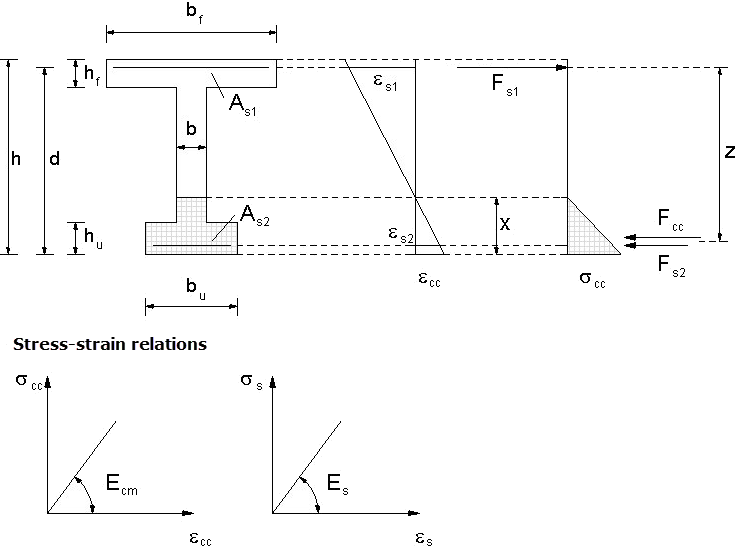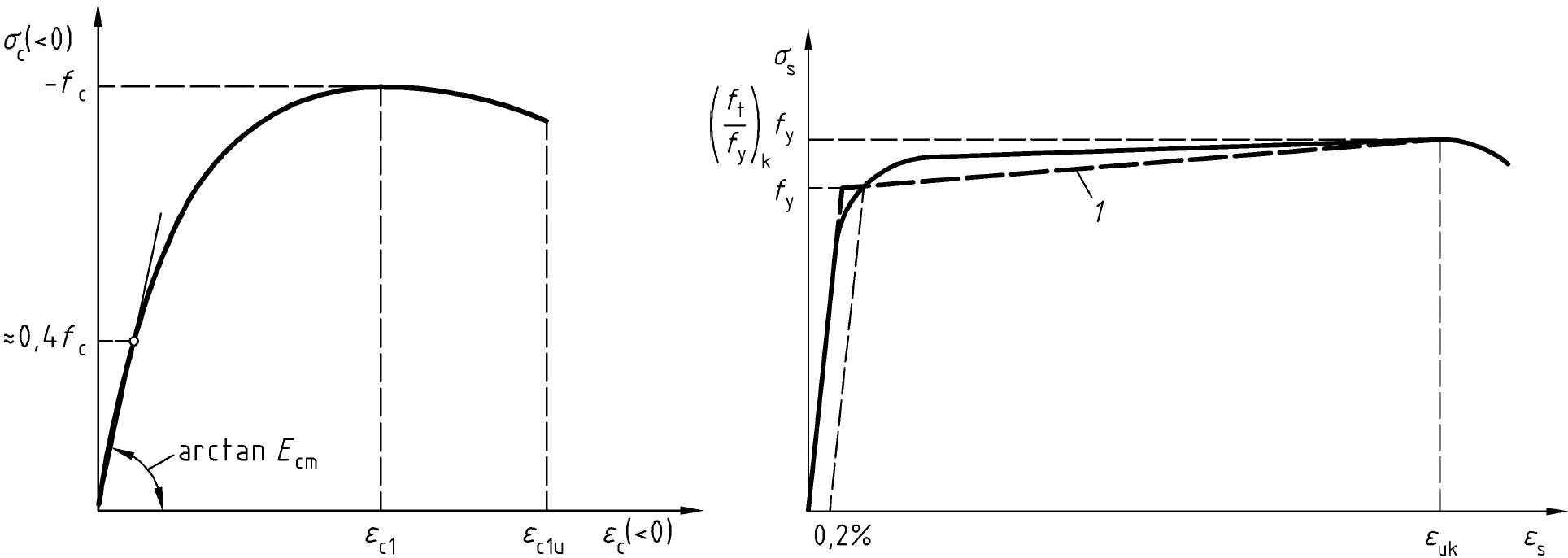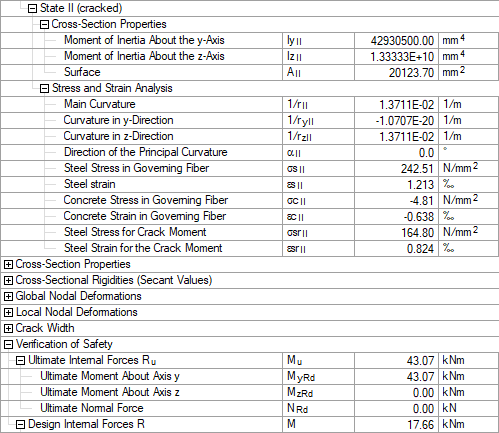# RF-CONCRETE Members – Online Manual Version 5

Online manuals, introductory examples, tutorials, and other documentation.

# 9.2.6.3 State II (cracked)

#### State II (cracked)

Cross-section properties in cracked state (state II)

In contrast to the cross-section properties in the uncracked state (state I), the cross-section properties in state II (cracked sections) are quite difficult to determine manually. Determining the strain distribution (general case: ε0 + (1/r)y ⋅ y + (1/r)z ⋅ z) for a particular action constellation with the stress-strain relations defined in the standards for nonlinear methods already represents a problem. For further information, refer to the corresponding literature .

Steel stress σsrII and steel strain εsrII for crack moment

To determine the stresses and strains for crack formation, we can normally make simplified assumptions (linear elastic material rules). We can justify this approach by the fact that the ratio of stress to strain for concrete behaves nearly linearly up to a stress of σc ≅ 0.4 ⋅ fc. For reinforcing steel, we can roughly assume this fact until yielding is reached anyway. Thus, if we have a structural component with a crack moment in the characteristic load level, we can calculate stresses and strains with sufficient accuracy using these simplified approaches.

Without an acting axial force, the solution for a triangular compression zone leads to a quadratic equation (with axial force: cubic equation) when calculating the neutral axis depth x (height of compression zone). Due to the assumed linearity of stresses and strains, the neutral axis depth is decoupled from the applied moment.Figure 9.24 Relations for calculating the stresses and strains for characteristic loads
Calculation of neutral axis depth xII

Moment of inertia

Stresses for crack internal forces

Steel strain for crack internal forces

Steel stress and concrete stress for available moment

A simplified calculation of stresses and strains, as was done for the crack moment, cannot be applied without due consideration. The stresses and strains for the effective moment M = 17.64 kNm required for the calculation of the curvatures and stiffnesses are determined in a comparative calculation using the exact stress-strain curves for concrete and reinforcing steel according to EN 1992-1-1, Figure 3.2 or 3.3.Figure 9.25 Relations for calculating stresses and strains for characteristic loads according to 

The accurate calculation of stresses in the cracked state is performed by means of a third party application used for exact stress integration, leading to the following results for M = 17.64 kNm:

• σs1,II = 242.27 N/mm2
• σs2,II = −59.07 N/mm2
• εs1,II = 1.211 ‰
• εs2,II = −0.638 ‰
• εc,II = −0.6378 ‰
Results of RF-CONCRETE MembersFigure 9.26 Detailed results for state II
Literatur
  Eurocode 2: Design of concrete structures - Part 1-1: General rules and rules for buildings; EN 1992-1-1:2011-01  Deutscher Ausschuss für Stahlbetonbau (Hrsg.) Heft 415 – Programmgesteuerte Berechnung beliebiger Massivbauquerschnitte unter zweiachsiger Biegung mit Längskraft. Beuth Verlag GmbH, Berlin, 1990.